DLI ISO32 Object Model Reference
20210317T210828Z
Meter server object
 object meter/ Object representing the meter server state and configuration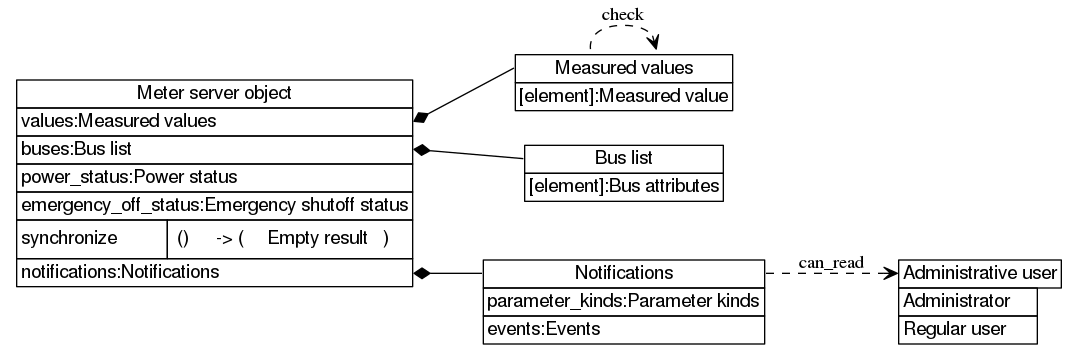This object contains the following items:

Sample `requests` read command:

```print(requests.get('http://192.168.0.100/restapi/meter/',auth=auth,headers=headers).json())
```

```<...>
```

Sample JS `request` write command:

```request.put({url:"meter/",body:<...>});
```

# Measured values

 map meter/values/ Values measured by meters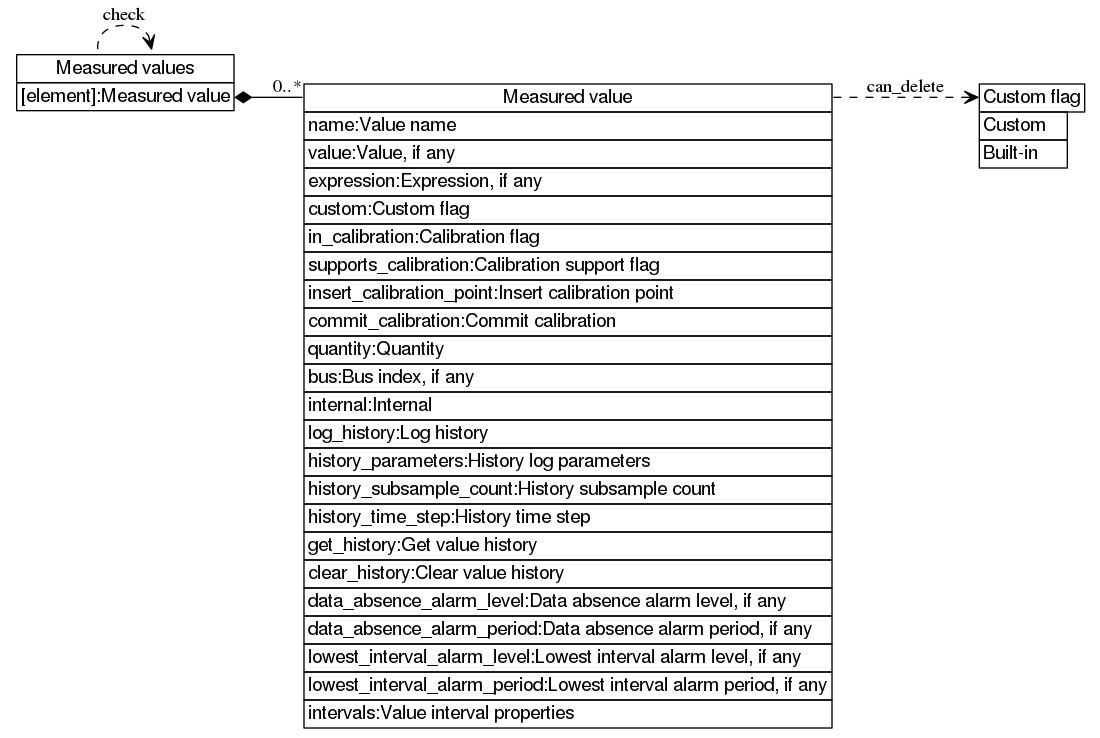This map contains Measured value elements.

Sample `requests` measured value creation command:

```requests.put('http://192.168.0.100/restapi/meter/values/vcc_voltage/',auth=auth,headers=headers,json={'intervals': [{'lower_bound_bottom': 325.83333333333, 'alarm_level': None, 'lower_bound_top': 336.66666666667, 'alarm_period': 1}, {'lower_bound_bottom': 335, 'alarm_level': 2, 'lower_bound_top': 344.16666666667, 'alarm_period': 2}, {'lower_bound_bottom': 349.16666666667, 'alarm_level': None, 'lower_bound_top': 350.83333333333, 'alarm_period': 1}, {'lower_bound_bottom': 375, 'alarm_level': 1, 'lower_bound_top': 390, 'alarm_period': None}], 'in_calibration': True, 'expression': '7.4', 'history_subsample_count': 15, 'data_absence_alarm_level': 6, 'value': False, 'log_history': False, 'history_time_step': 30, 'history_parameters': [[300, 86400], [3600, 1209600], [86400, 31536000]], 'lowest_interval_alarm_period': 3, 'data_absence_alarm_period': None, 'quantity': 'voltage', 'name': 'DC voltage', 'supports_calibration': False, 'internal': False, 'lowest_interval_alarm_level': 1, 'bus': None})
```

Sample JS `request` read command:

```request.get({url:"meter/values/"},function(error,response,body) {console.log(body)});
```

```<...>
```

## Measured value

 object meter/values/S/ Value measured by meter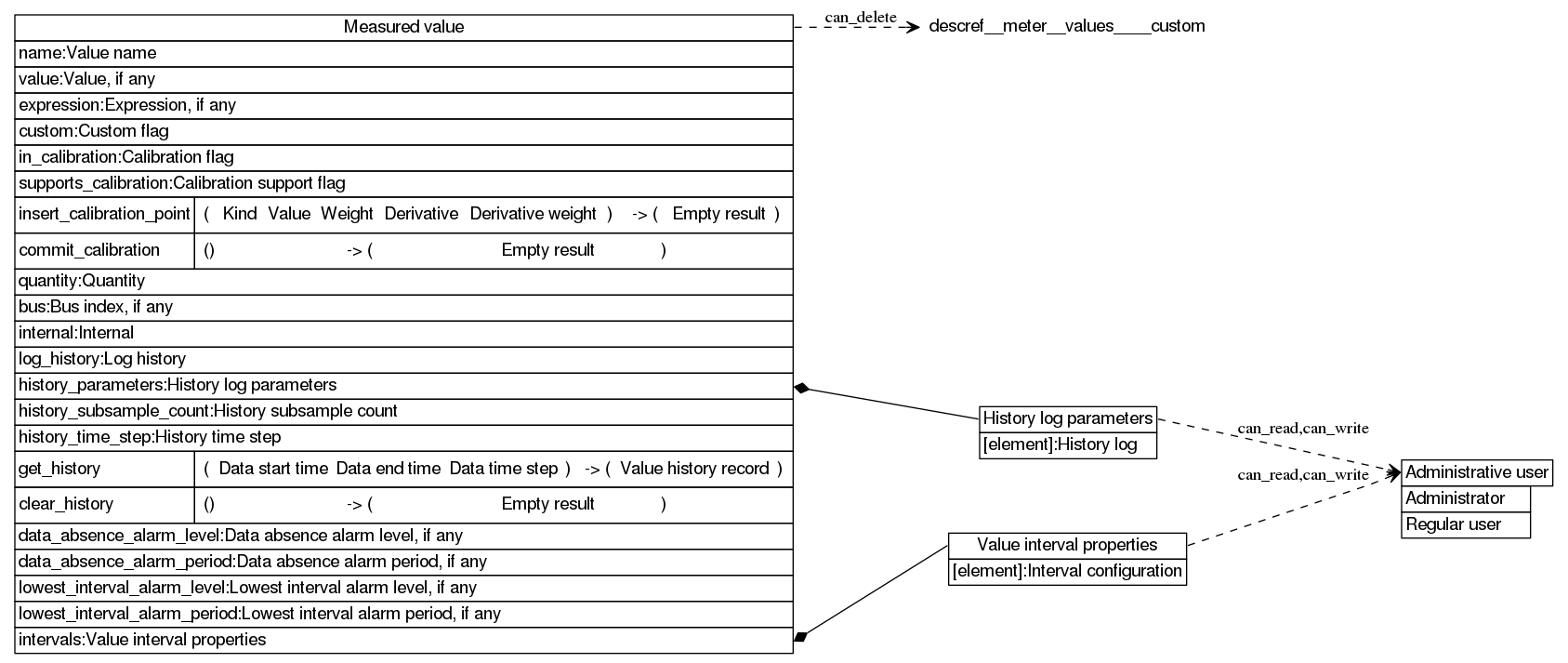The value is subject to the following constraints: not index of the value matches ^(|​.*|﻿.*)\$

Removing the object from its parent collection is denied if not custom flag

This object contains the following items:

Sample JS `request` write command (note that `_measured_value` is a variable):

```request.put({url:"meter/values/"+_measured_value+"/",body:<...>});
```

Sample JS `request` deletion command (note that `_measured_value` is a variable):

```request.delete({url:"meter/values/"+_measured_value+"/"});
```

# Measured value: Value name

 string meter/values/S/name/ Name of the measured value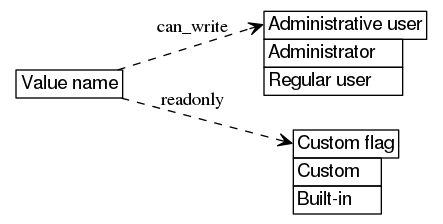Changing the value is denied if not administrative user

This string does not support direct modification if not custom flag.

This string represents a value in persistent storage.

Sample JS `request` write command (note that `_measured_value` is a variable):

```request.put({url:"meter/values/"+_measured_value+"/name/",body:"DC voltage"});
```

Sample CLI read command (note that `measured_value` is a variable):

```uom get "meter/values/\${measured_value}/name"
```

```"DC voltage"
```

# Measured value: Value, if any

 sum (variant) meter/values/S/value/ Current value measured by meter, if any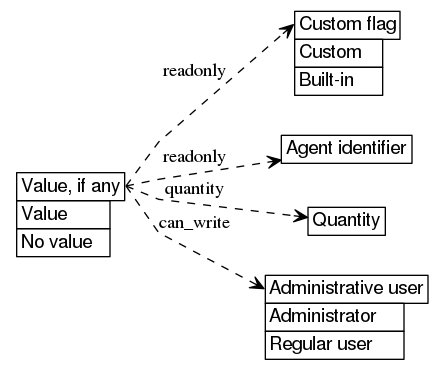Changing the value is denied if not administrative user

This sum does not support direct modification if all of the following is true:

This sum can change as if by itself or indirectly because of other actions.

Changes to this sum may have an effect besides setting value.

This sum can assume the following values:

 number Value Current value measured by meter constant false No value Indication that measurement is currently not being carried out

Sample CLI read command (note that `measured_value` is a variable):

```uom get "meter/values/\${measured_value}/value"
```

```5
```

Sample JS `request` write command (note that `_measured_value` is a variable):

```request.put({url:"meter/values/"+_measured_value+"/value/",body:276});
```

# Measured value: Expression, if any

 sum (optional string) meter/values/S/expression/ Expression governing the value, if any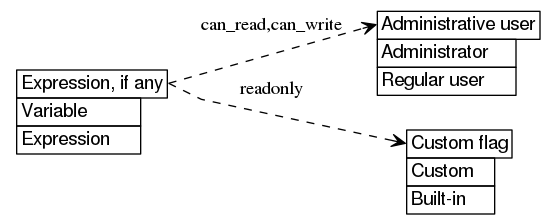Retrieving the value is denied if not administrative user

Changing the value is denied if not administrative user

This sum does not support direct modification if not custom flag.

This sum represents a value in persistent storage.

This sum can change as if by itself or indirectly because of other actions.

This sum can assume the following values:

 constant null Variable The value can be modified arbitrarily string Expression Expression governing the value

Sample CLI write command (note that `measured_value` is a variable):

```uom set "meter/values/\${measured_value}/expression" "null"
```

Sample `uom` library write command (note that `_measured_value` is a variable):

```uom.meter.values[_measured_value].expression=null
```

# Measured value: Custom flag

 sum (boolean) meter/values/S/custom/ Flag indicating whether the value is custom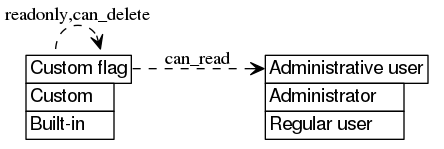The value is unconditionally set to `true` on creation and doesn't have to be supplied (if it is, it must match)

Retrieving the value is denied if not administrative user

This sum does not support direct modification.

This sum represents a value in persistent storage.

This sum can assume the following values:

 constant true Custom The value is custom and can be modified freely constant false Built-in The value is built-in and only the hardware can modify it

Sample CLI read command (note that `measured_value` is a variable):

```uom get "meter/values/\${measured_value}/custom"
```

```[]
```

Sample `curl` read command (note that `:measured_value` is a URL template argument):

```curl --digest -u admin:1234 -H "Accept: application/json" "http://192.168.0.100/restapi/meter/values/:measured_value/custom/"
```

```[]
```

# Measured value: Calibration flag

 sum (boolean) meter/values/S/in_calibration/ Flag indicating whether the value is being calibrated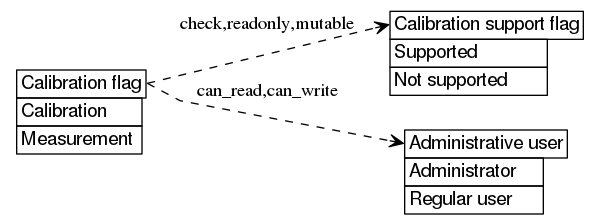The value is subject to the following constraints: any of the following is true:

Retrieving the value is denied if not administrative user

Changing the value is denied if not administrative user

This sum does not support direct modification if not calibration support flag.

This sum can change as if by itself or indirectly because of other actions if calibration support flag.

This sum can assume the following values:

 constant true Calibration The value is being calibrated (it can be updated to match external reference) constant false Measurement The value is being measured

Sample JS `request` read command (note that `_measured_value` is a variable):

```request.get({url:"meter/values/"+_measured_value+"/in_calibration/"},function(error,response,body) {console.log(body)});
```

```true
```

Sample `curl` write command (note that `:measured_value` is a URL template argument):

```curl --digest -u admin:1234 -H "X-CSRF: x" -X PUT -H "Content-type: application/json" -H "Accept: application/json" --data-binary "true" "http://192.168.0.100/restapi/meter/values/:measured_value/in_calibration/"
```

# Measured value: Calibration support flag

 sum (boolean) meter/values/S/supports_calibration/ Flag indicating whether calibrating the value is supported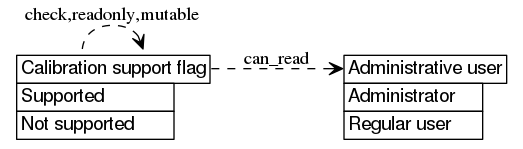Retrieving the value is denied if not administrative user

This sum does not support direct modification.

This sum represents a value in persistent storage.

This sum can assume the following values:

 constant true Supported The value can be calibrated constant false Not supported There is no support for calibrating the value, or it does not make sense to

Sample JS `request` read command (note that `_measured_value` is a variable):

```request.get({url:"meter/values/"+_measured_value+"/supports_calibration/"},function(error,response,body) {console.log(body)});
```

```false
```

Sample `curl` read command (note that `:measured_value` is a URL template argument):

```curl --digest -u admin:1234 -H "Accept: application/json" "http://192.168.0.100/restapi/meter/values/:measured_value/supports_calibration/"
```

```true
```

# Measured value: Insert calibration point

 call meter/values/S/insert_calibration_point/ Specify custom calibration point, possibly overriding measurements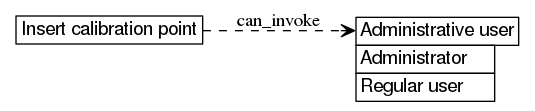Performing the function call is denied if any of the following is true:

The call accepts the following arguments:

1. Kind: Standard, Identity or Current
2. Value: number
3. Weight: number
4. Derivative: number
5. Derivative weight: number

The call returns the following results:

1. Empty result: null

Sample JS `request` invocation (note that `_measured_value` is a variable):

```request.post({url:"meter/values/"+_measured_value+"/insert_calibration_point/",body:["current",92,7.4,-4.3,8.5]});
```

```(no output)
```

Sample CLI invocation (note that `measured_value` is a variable):

```uom invoke "meter/values/\${measured_value}/insert_calibration_point" "\"current\"" "7" "9.7" "3.7" "8.9"
```

```(no output)
```

## Kind

 sum (enumerated string) meter/values/S/insert_calibration_point/arguments: 1/ Kind of calibration point

This sum does not correspond to an actual resource.

This sum can assume the following values:

 constant "standard" Standard The point supplies the calibrated value constant "identity" Identity Assume the calibration function is the identity in point constant "current" Current Assume the calibration function keeps current value in point

## Value

 number meter/values/S/insert_calibration_point/arguments: 2/ Calibrated value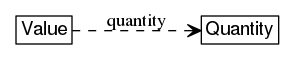The value represents quantity.

This number does not correspond to an actual resource.

## Weight

 number meter/values/S/insert_calibration_point/arguments: 3/ Weight of the calibration point

The value represents ratio, which is dimensionless.

To convert to percents, multiply by 100.

To convert from percents, divide by 100.

The value is subject to the following constraints: the value is greater or equal to `0`

This number does not correspond to an actual resource.

## Derivative

 number meter/values/S/insert_calibration_point/arguments: 4/ Derivative value in the calibration point

The value represents ratio, which is dimensionless.

To convert to percents, multiply by 100.

To convert from percents, divide by 100.

This number does not correspond to an actual resource.

## Derivative weight

 number meter/values/S/insert_calibration_point/arguments: 5/ Weight of derivative value in the calibration point

The value represents ratio, which is dimensionless.

To convert to percents, multiply by 100.

To convert from percents, divide by 100.

The value is subject to the following constraints: the value is greater or equal to `0`

This number does not correspond to an actual resource.

## Empty result

 constant null meter/values/S/insert_calibration_point/results: 1/ No additional information available

This constant does not correspond to an actual resource.

# Measured value: Commit calibration

 call meter/values/S/commit_calibration/ Save calibration data to persistent storage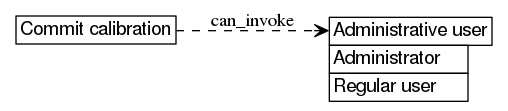Performing the function call is denied if any of the following is true:

The call has no arguments (supply empty tuple).

The call returns the following results:

1. Empty result: null

Sample `uom` library invocation (note that `_measured_value` is a variable):

```uom.meter.values[_measured_value].commit_calibration()
```

```(no output)
```

Sample `requests` invocation (note that `_measured_value` is a variable):

```requests.post('http://192.168.0.100/restapi/meter/values/'+_measured_value+'/commit_calibration/',auth=auth,headers=headers,json={})
```

```(no output)
```

## Empty result

 constant null meter/values/S/commit_calibration/results: 1/ No additional information available

This constant does not correspond to an actual resource.

# Measured value: Quantity

 string meter/values/S/quantity/ Quantity of the value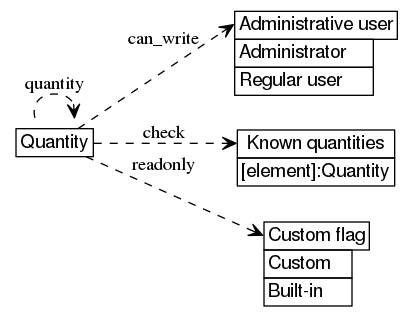The value is subject to the following constraints: known quantities[the value]

Changing the value is denied if not administrative user

This string does not support direct modification if not custom flag.

This string represents a value in persistent storage.

Sample `requests` read command (note that `_measured_value` is a variable):

```print(requests.get('http://192.168.0.100/restapi/meter/values/'+_measured_value+'/quantity/',auth=auth,headers=headers).json())
```

```'voltage'
```

Sample JS `request` write command (note that `_measured_value` is a variable):

```request.put({url:"meter/values/"+_measured_value+"/quantity/",body:"voltage"});
```

# Measured value: Bus index, if any

 sum (optional number) meter/values/S/bus/ Index of bus being measured, if any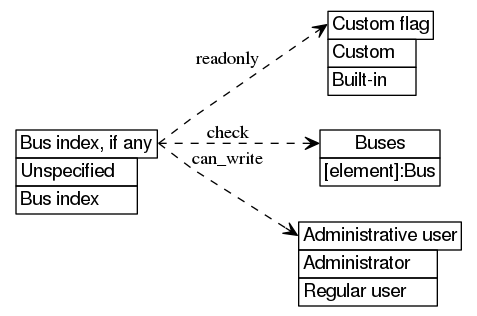Changing the value is denied if not administrative user

This sum does not support direct modification if not custom flag.

This sum represents a value in persistent storage.

This sum can assume the following values:

 constant null Unspecified The value is not related to a bus number Bus index Index of bus being measured buses[the value]

Sample JS `request` read command (note that `_measured_value` is a variable):

```request.get({url:"meter/values/"+_measured_value+"/bus/"},function(error,response,body) {console.log(body)});
```

```null
```

Sample `curl` write command (note that `:measured_value` is a URL template argument):

```curl --digest -u admin:1234 -H "X-CSRF: x" -X PUT -H "Content-type: application/json" -H "Accept: application/json" --data-binary "1" "http://192.168.0.100/restapi/meter/values/:measured_value/bus/"
```

# Measured value: Internal

 sum (boolean) meter/values/S/internal/ Flag indicating whether this value is internal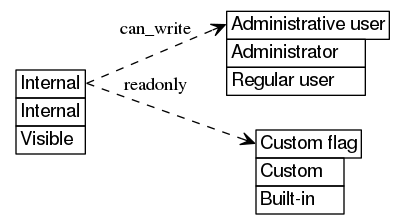Changing the value is denied if not administrative user

This sum does not support direct modification if not custom flag.

This sum represents a value in persistent storage.

This sum can assume the following values:

 constant true Internal The value is internal and should not matter to users constant false Visible The value should be visible to users

Sample `curl` write command (note that `:measured_value` is a URL template argument):

```curl --digest -u admin:1234 -H "X-CSRF: x" -X PUT -H "Content-type: application/json" -H "Accept: application/json" --data-binary "true" "http://192.168.0.100/restapi/meter/values/:measured_value/internal/"
```

Sample JS `request` write command (note that `_measured_value` is a variable):

```request.put({url:"meter/values/"+_measured_value+"/internal/",body:true});
```

# Measured value: Log history

 sum (boolean) meter/values/S/log_history/ Flag indicating whether the value should be logged to the history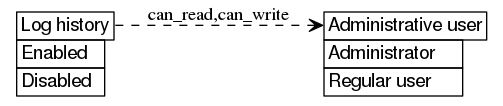Retrieving the value is denied if not administrative user

Changing the value is denied if not administrative user

This sum represents a value in persistent storage.

This sum can assume the following values:

 constant true Enabled The feature is enabled constant false Disabled The feature is disabled

Sample `curl` write command (note that `:measured_value` is a URL template argument):

```curl --digest -u admin:1234 -H "X-CSRF: x" -X PUT -H "Content-type: application/json" -H "Accept: application/json" --data-binary "false" "http://192.168.0.100/restapi/meter/values/:measured_value/log_history/"
```

Sample `requests` read command (note that `_measured_value` is a variable):

```print(requests.get('http://192.168.0.100/restapi/meter/values/'+_measured_value+'/log_history/',auth=auth,headers=headers).json())
```

```True
```

# Measured value: History log parameters

 array meter/values/S/history_parameters/ Configuration of history logs sampling intervals and timespans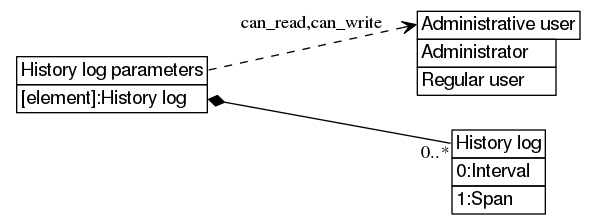Retrieving the value is denied if not administrative user

Changing the value is denied if not administrative user

This array is composite, i.e. is modified as a whole.

This array represents a value in persistent storage.

This array can change as if by itself or indirectly because of other actions.

This array contains History log elements.

Sample `requests` read command (note that `_measured_value` is a variable):

```print(requests.get('http://192.168.0.100/restapi/meter/values/'+_measured_value+'/history_parameters/',auth=auth,headers=headers).json())
```

```[[300, 86400], [3600, 1209600], [86400, 31536000]]
```

Sample `uom` library read command (note that `_measured_value` is a variable):

```print(uom.dump(uom.meter.values[_measured_value].history_parameters))
```

```{{300,86400},{3600,1209600},{86400,31536000}}
```

## History log

 tuple meter/values/S/history_parameters/N/ History log settings

This tuple is a part of a composite value which is modified as a whole.

This tuple contains the following items:

 1 Interval 2 Span

Sample CLI read command (note that `measured_value` and `history_log_index` are variables):

```uom get "meter/values/\${measured_value}/history_parameters/\${history_log_index}"
```

```[3600,1209600]
```

Sample `curl` deletion command (note that `:measured_value` and `:history_log_index` are URL template arguments):

```curl --digest -u admin:1234 -H "X-CSRF: x" -X DELETE -H "Accept: application/json" "http://192.168.0.100/restapi/meter/values/:measured_value/history_parameters/:history_log_index/"
```

### Interval

 number meter/values/S/history_parameters/N/1/ Time interval between two consecutive history samples (changes will trigger history rebuilding and may cause adjustments to time span)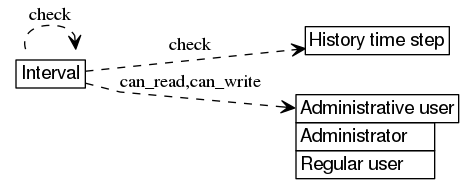The value represents time, the standard unit of which is the second (s).

The value is subject to the following constraints: all of the following is true:

Retrieving the value is denied if not administrative user

Changing the value is denied if not administrative user

This number is a part of a composite value which is modified as a whole.

This number represents a value in persistent storage.

This number can change as if by itself or indirectly because of other actions.

Sample CLI read command (note that `measured_value` and `history_log_index` are variables):

```uom get "meter/values/\${measured_value}/history_parameters/\${history_log_index}/0"
```

```3600
```

Sample JS `request` read command (note that `_measured_value` and `_history_log_index` are variables):

```request.get({url:"meter/values/"+_measured_value+"/history_parameters/"+_history_log_index.toString()+"/0/"},function(error,response,body) {console.log(body)});
```

```86400
```

### Span

 number meter/values/S/history_parameters/N/2/ Time span covered by history fragment (changes will trigger history rebuilding)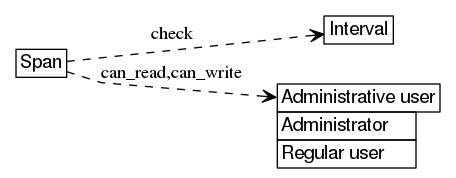The value represents time, the standard unit of which is the second (s).

The value is subject to the following constraints: all of the following is true:

Retrieving the value is denied if not administrative user

Changing the value is denied if not administrative user

This number is a part of a composite value which is modified as a whole.

This number represents a value in persistent storage.

This number can change as if by itself or indirectly because of other actions.

Sample `curl` write command (note that `:measured_value` and `:history_log_index` are URL template arguments):

```curl --digest -u admin:1234 -H "X-CSRF: x" -X PUT -H "Content-type: application/json" -H "Accept: application/json" --data-binary "31536000" "http://192.168.0.100/restapi/meter/values/:measured_value/history_parameters/:history_log_index/1/"
```

Sample `requests` write command (note that `_measured_value` and `_history_log_index` are variables):

```requests.put('http://192.168.0.100/restapi/meter/values/'+_measured_value+'/history_parameters/'+str(_history_log_index)+'/1/',auth=auth,headers=headers,json=1209600)
```

# Measured value: History subsample count

 number meter/values/S/history_subsample_count/ Number of measurements per history sample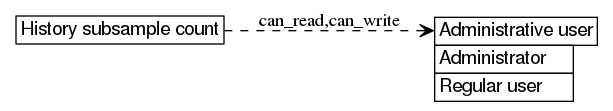The value represents count, the standard unit of which is the time (times).

The value is subject to the following constraints: all of the following is true:

Retrieving the value is denied if not administrative user

Changing the value is denied if not administrative user

This number represents a value in persistent storage.

Sample JS `request` read command (note that `_measured_value` is a variable):

```request.get({url:"meter/values/"+_measured_value+"/history_subsample_count/"},function(error,response,body) {console.log(body)});
```

```22
```

Sample CLI write command (note that `measured_value` is a variable):

```uom set "meter/values/\${measured_value}/history_subsample_count" "25"
```

# Measured value: History time step

 number meter/values/S/history_time_step/ Minimal amount of time between saved history samples (changes will trigger history rebuilding and may cause adjustments to history_parameters)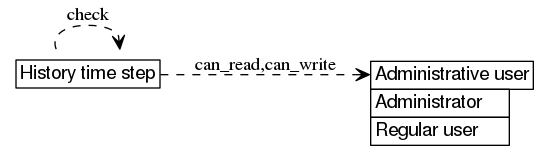The value represents time, the standard unit of which is the second (s).

The value is subject to the following constraints: the value is greater than `0`

Retrieving the value is denied if not administrative user

Changing the value is denied if not administrative user

This number represents a value in persistent storage.

Sample JS `request` read command (note that `_measured_value` is a variable):

```request.get({url:"meter/values/"+_measured_value+"/history_time_step/"},function(error,response,body) {console.log(body)});
```

```13
```

Sample `curl` write command (note that `:measured_value` is a URL template argument):

```curl --digest -u admin:1234 -H "X-CSRF: x" -X PUT -H "Content-type: application/json" -H "Accept: application/json" --data-binary "29" "http://192.168.0.100/restapi/meter/values/:measured_value/history_time_step/"
```

# Value history record: Value history

 array meter/values/S/get_history/results: 1/3/ Historical data points

This array does not correspond to an actual resource.

This array contains Data point, if any elements.

## Data point, if any

 sum (variant) meter/values/S/get_history/results: 1/3/N/ Historical data point, if any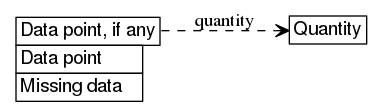This sum does not correspond to an actual resource.

This sum can assume the following values:

 number Data point Historical data point, whose corresponding time is determined by array position constant false Missing data Indication that data for the time determined by array position could not be found

# Measured value: Get value history

 call meter/values/S/get_history/ Get history of values over a specific time rangePerforming the function call is denied if not administrative user

The call accepts the following arguments:

1. Data start time: number
2. Data end time: number
3. Data time step: number

The call returns the following results:

1. Value history record: (number,number,array of "Data point or Missing data")

Sample CLI invocation (note that `measured_value` is a variable):

```uom invoke "meter/values/\${measured_value}/get_history" "1573568048" "1573654448" "86400"
```

```[1573516800,86400,]
```

Sample `curl` invocation (note that `:measured_value` is a URL template argument):

```curl --digest -u admin:1234 -H "X-CSRF: x" -H "Content-type: application/json" -H "Accept: application/json" --data-binary "[1516780919,1516781099,60]" "http://192.168.0.100/restapi/meter/values/:measured_value/get_history/"
```

```[1516780860,60,[322,312,false]]
```

## Data start time

 number meter/values/S/get_history/arguments: 1/ Requested timestamp of the first data point to retrieve

The value represents time, the standard unit of which is the second (s).

This number does not correspond to an actual resource.

## Data end time

 number meter/values/S/get_history/arguments: 2/ Requested timestamp of the last data point to retrieve

The value represents time, the standard unit of which is the second (s).

This number does not correspond to an actual resource.

## Data time step

 number meter/values/S/get_history/arguments: 3/ Requested time step between succeeding data points

The value represents time, the standard unit of which is the second (s).

The value is subject to the following constraints: the value is greater or equal to `0`

This number does not correspond to an actual resource.

## Value history record

 tuple meter/values/S/get_history/results: 1/ Historical measured values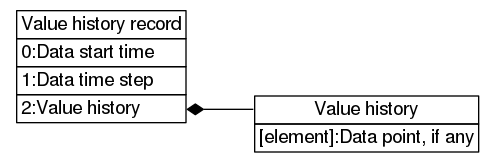This tuple does not correspond to an actual resource.

This tuple contains the following items:

### Data start time

 number meter/values/S/get_history/results: 1/1/ Timestamp of the first retrieved historical data point (may differ from requested start timestamp)

The value represents time, the standard unit of which is the second (s).

This number does not correspond to an actual resource.

### Data time step

 number meter/values/S/get_history/results: 1/2/ Time step between succeeding retrieved historical data points (may differ from requested time step)

The value represents time, the standard unit of which is the second (s).

The value is subject to the following constraints: the value is greater or equal to `0`

This number does not correspond to an actual resource.

# Measured value: Clear value history

 call meter/values/S/clear_history/ Clear the complete history of values, and reset value to zero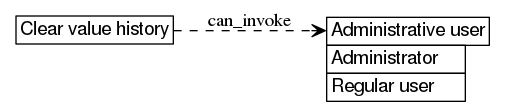Performing the function call is denied if not administrative user

The call has no arguments (supply empty tuple).

The call returns the following results:

1. Empty result: null

Sample CLI invocation (note that `measured_value` is a variable):

```uom invoke "meter/values/\${measured_value}/clear_history"
```

```(no output)
```

Sample `uom` library invocation (note that `_measured_value` is a variable):

```uom.meter.values[_measured_value].clear_history()
```

```(no output)
```

## Empty result

 constant null meter/values/S/clear_history/results: 1/ No additional information available

This constant does not correspond to an actual resource.

# Measured value: Data absence alarm level, if any

 sum (optional number) meter/values/S/data_absence_alarm_level/ Alarm level for absence of data, if any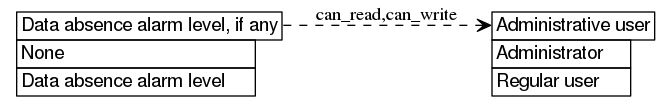Retrieving the value is denied if not administrative user

Changing the value is denied if not administrative user

This sum represents a value in persistent storage.

This sum can assume the following values:

 constant null None No alarm is generated on absence of data number Data absence alarm level Alarm level for absence of data

Sample CLI read command (note that `measured_value` is a variable):

```uom get "meter/values/\${measured_value}/data_absence_alarm_level"
```

```6
```

Sample `requests` write command (note that `_measured_value` is a variable):

```requests.put('http://192.168.0.100/restapi/meter/values/'+_measured_value+'/data_absence_alarm_level/',auth=auth,headers=headers,json=3)
```

# Measured value: Data absence alarm period, if any

 sum (optional number) meter/values/S/data_absence_alarm_period/ The time interval after which data absence alarm events should be repeated, if any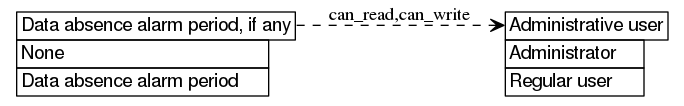Retrieving the value is denied if not administrative user

Changing the value is denied if not administrative user

This sum represents a value in persistent storage.

This sum can assume the following values:

 constant null None The data absence alarm event is generated only once on data loss and is not repeated number Data absence alarm period The time interval after which data absence alarm events should be repeated the value is greater than `0`

Sample `uom` library read command (note that `_measured_value` is a variable):

```print(uom.dump(uom.meter.values[_measured_value].data_absence_alarm_period))
```

```null
```

Sample `requests` read command (note that `_measured_value` is a variable):

```print(requests.get('http://192.168.0.100/restapi/meter/values/'+_measured_value+'/data_absence_alarm_period/',auth=auth,headers=headers).json())
```

```None
```

# Measured value: Lowest interval alarm level, if any

 sum (optional number) meter/values/S/lowest_interval_alarm_level/ Alarm level for the lowest value interval, if any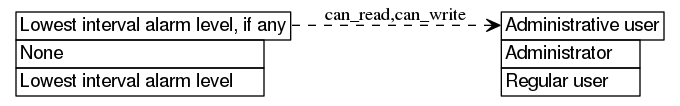Retrieving the value is denied if not administrative user

Changing the value is denied if not administrative user

This sum represents a value in persistent storage.

This sum can assume the following values:

 constant null None No alarm is generated for the lowest value interval number Lowest interval alarm level Alarm level for the lowest value interval

Sample `uom` library write command (note that `_measured_value` is a variable):

```uom.meter.values[_measured_value].lowest_interval_alarm_level=1
```

Sample CLI read command (note that `measured_value` is a variable):

```uom get "meter/values/\${measured_value}/lowest_interval_alarm_level"
```

```2
```

# Measured value: Lowest interval alarm period, if any

 sum (optional number) meter/values/S/lowest_interval_alarm_period/ The time interval after which lowest interval alarm events should be repeated, if any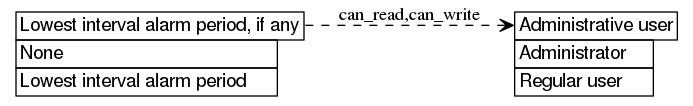Retrieving the value is denied if not administrative user

Changing the value is denied if not administrative user

This sum represents a value in persistent storage.

This sum can assume the following values:

 constant null None The alarm event is generated only once at lowest interval entry and is not repeated while the value is in the interval number Lowest interval alarm period The time interval after which lowest interval alarm events should be repeated the value is greater than `0`

Sample CLI write command (note that `measured_value` is a variable):

```uom set "meter/values/\${measured_value}/lowest_interval_alarm_period" "4"
```

Sample `uom` library read command (note that `_measured_value` is a variable):

```print(uom.dump(uom.meter.values[_measured_value].lowest_interval_alarm_period))
```

```1
```

# Measured value: Value interval properties

 array meter/values/S/intervals/ Properties defining intervals of the value and their alarm levels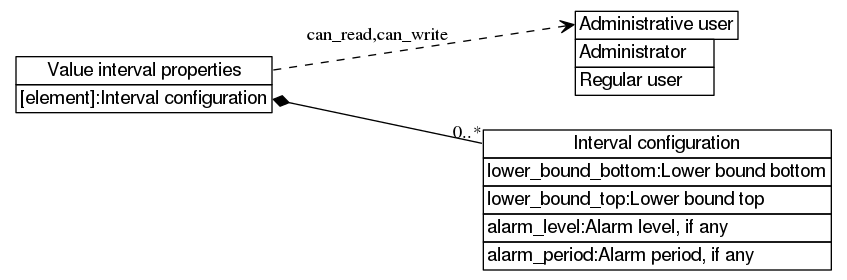Retrieving the value is denied if not administrative user

Changing the value is denied if not administrative user

This array is composite, i.e. is modified as a whole.

This array contains Interval configuration elements.

Sample `uom` library read command (note that `_measured_value` is a variable):

```print(uom.dump(uom.meter.values[_measured_value].intervals))
```

Sample JS `request` interval configuration creation command (note that `_measured_value` is a variable):

```request.post({url:"meter/values/"+_measured_value+"/intervals/",body:{"lower_bound_bottom":57.833333333333,"alarm_level":3,"lower_bound_top":58.916666666667,"alarm_period":null}});
```

## Interval configuration

 object meter/values/S/intervals/N/ Configuration of a value interval

This object is a part of a composite value which is modified as a whole.

This object contains the following items:

Sample `uom` library read command (note that `_measured_value` and `_interval_configuration_index` are variables):

```print(uom.dump(uom.meter.values[_measured_value].intervals[_interval_configuration_index]))
```

```{alarm_level=2,alarm_period=1,lower_bound_bottom=299,lower_bound_top=302}
```

Sample CLI deletion command (note that `measured_value` and `interval_configuration_index` are variables):

```uom remove "meter/values/\${measured_value}/intervals/\${interval_configuration_index}"
```

### Lower bound bottom

 number meter/values/S/intervals/N/lower_bound_bottom/ The bottom of the lower bound of this interval; value must be lower to be considered outside this interval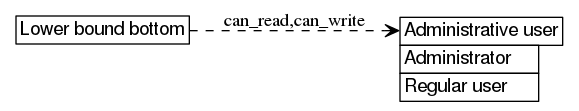Retrieving the value is denied if not administrative user

Changing the value is denied if not administrative user

This number is a part of a composite value which is modified as a whole.

This number represents a value in persistent storage.

Sample `requests` read command (note that `_measured_value` and `_interval_configuration_index` are variables):

```print(requests.get('http://192.168.0.100/restapi/meter/values/'+_measured_value+'/intervals/'+str(_interval_configuration_index)+'/lower_bound_bottom/',auth=auth,headers=headers).json())
```

```378
```

Sample `curl` write command (note that `:measured_value` and `:interval_configuration_index` are URL template arguments):

```curl --digest -u admin:1234 -H "X-CSRF: x" -X PUT -H "Content-type: application/json" -H "Accept: application/json" --data-binary "214" "http://192.168.0.100/restapi/meter/values/:measured_value/intervals/:interval_configuration_index/lower_bound_bottom/"
```

### Lower bound top

 number meter/values/S/intervals/N/lower_bound_top/ The top of the lower bound of this interval; value must be higher to be considered inside this interval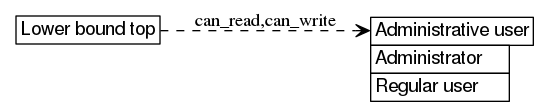Retrieving the value is denied if not administrative user

Changing the value is denied if not administrative user

This number is a part of a composite value which is modified as a whole.

This number represents a value in persistent storage.

Sample JS `request` read command (note that `_measured_value` and `_interval_configuration_index` are variables):

```request.get({url:"meter/values/"+_measured_value+"/intervals/"+_interval_configuration_index.toString()+"/lower_bound_top/"},function(error,response,body) {console.log(body)});
```

```209
```

Sample `curl` write command (note that `:measured_value` and `:interval_configuration_index` are URL template arguments):

```curl --digest -u admin:1234 -H "X-CSRF: x" -X PUT -H "Content-type: application/json" -H "Accept: application/json" --data-binary "10.09009009009" "http://192.168.0.100/restapi/meter/values/:measured_value/intervals/:interval_configuration_index/lower_bound_top/"
```

### Alarm level, if any

 sum (optional number) meter/values/S/intervals/N/alarm_level/ The level of importance assigned to this interval, if any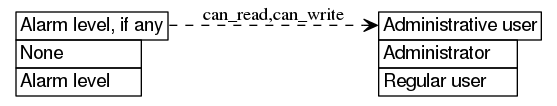Retrieving the value is denied if not administrative user

Changing the value is denied if not administrative user

This sum is a part of a composite value which is modified as a whole.

This sum represents a value in persistent storage.

This sum can assume the following values:

 constant null None No alarm is generated for this value interval number Alarm level The level of importance assigned to this interval

Sample JS `request` read command (note that `_measured_value` and `_interval_configuration_index` are variables):

```request.get({url:"meter/values/"+_measured_value+"/intervals/"+_interval_configuration_index.toString()+"/alarm_level/"},function(error,response,body) {console.log(body)});
```

```1
```

Sample `curl` read command (note that `:measured_value` and `:interval_configuration_index` are URL template arguments):

```curl --digest -u admin:1234 -H "Accept: application/json" "http://192.168.0.100/restapi/meter/values/:measured_value/intervals/:interval_configuration_index/alarm_level/"
```

```1
```

### Alarm period, if any

 sum (optional number) meter/values/S/intervals/N/alarm_period/ The time interval after which alarm events should be repeated, if anyRetrieving the value is denied if not administrative user

Changing the value is denied if not administrative user

This sum is a part of a composite value which is modified as a whole.

This sum represents a value in persistent storage.

This sum can assume the following values:

 constant null None The alarm event is generated only once at interval entry and is not repeated while the value is in the interval number Alarm period The time interval after which alarm events should be repeated the value is greater than `0`

Sample `uom` library write command (note that `_measured_value` and `_interval_configuration_index` are variables):

```uom.meter.values[_measured_value].intervals[_interval_configuration_index].alarm_period=3
```

Sample `curl` write command (note that `:measured_value` and `:interval_configuration_index` are URL template arguments):

```curl --digest -u admin:1234 -H "X-CSRF: x" -X PUT -H "Content-type: application/json" -H "Accept: application/json" --data-binary "1" "http://192.168.0.100/restapi/meter/values/:measured_value/intervals/:interval_configuration_index/alarm_period/"
```

# Bus list

 array meter/buses/ Attributes for buses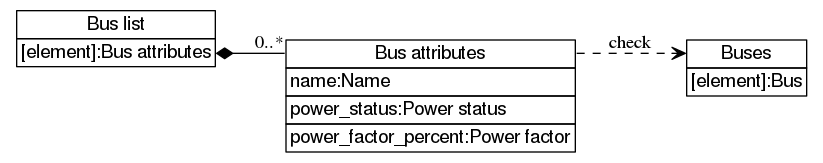This array does not support direct modification.

This array contains Bus attributes elements.

Creating elements in this collection is not supported.

Sample `curl` read command:

```curl --digest -u admin:1234 -H "Accept: application/json" "http://192.168.0.100/restapi/meter/buses/"
```

```[{"power_status":true,"name":"Bus A","power_factor_percent":100},{"power_status":false,"name":"Bus B","power_factor_percent":100}]
```

Sample JS `request` read command:

```request.get({url:"meter/buses/"},function(error,response,body) {console.log(body)});
```

```[{"power_status":true,"name":"Bus A","power_factor_percent":100},{"power_status":false,"name":"Bus B","power_factor_percent":100}]
```

# Bus list: Bus attributes

 object meter/buses/N/ Attributes for a bus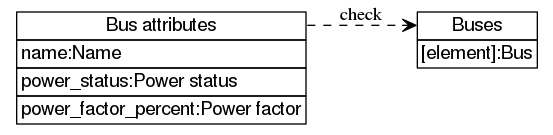The value is subject to the following constraints: buses[index of the value]

This object contains the following items:

Sample `uom` library write command (note that `_bus_attributes_index` is a variable):

```uom.meter.buses[_bus_attributes_index]={name="Bus B",power_factor_percent=100,power_status=false}
```

Sample CLI read command (note that `bus_attributes_index` is a variable):

```uom get "meter/buses/\${bus_attributes_index}"
```

```{"power_status":true,"name":"Bus A","power_factor_percent":100}
```

## Name

 string meter/buses/N/name/ User-visible name of this bus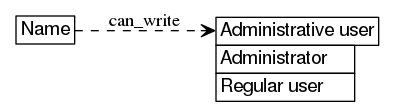Changing the value is denied if not administrative user

This string represents a value in persistent storage.

Sample CLI write command (note that `bus_attributes_index` is a variable):

```uom set "meter/buses/\${bus_attributes_index}/name" "\"Bus A\""
```

Sample CLI read command (note that `bus_attributes_index` is a variable):

```uom get "meter/buses/\${bus_attributes_index}/name"
```

```"Bus B"
```

## Power status

 sum (boolean) meter/buses/N/power_status/ Status of bus power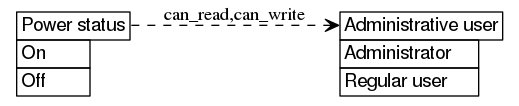Retrieving the value is denied if not administrative user

Changing the value is denied if not administrative user

This sum does not support direct modification.

This sum can change as if by itself or indirectly because of other actions.

This sum can assume the following values:

 constant true On Bus is powered constant false Off Bus is not powered

Sample `uom` library read command (note that `_bus_attributes_index` is a variable):

```print(uom.dump(uom.meter.buses[_bus_attributes_index].power_status))
```

```false
```

Sample `curl` read command (note that `:bus_attributes_index` is a URL template argument):

```curl --digest -u admin:1234 -H "Accept: application/json" "http://192.168.0.100/restapi/meter/buses/:bus_attributes_index/power_status/"
```

```true
```

## Power factor

 number meter/buses/N/power_factor_percent/ Power factor for this bus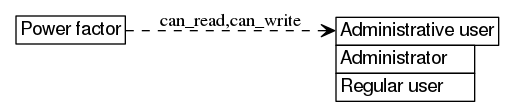The value is subject to the following constraints: all of the following is true:

• the value is greater or equal to `0`
• the value is less or equal to `100`

Retrieving the value is denied if not administrative user

Changing the value is denied if not administrative user

This number represents a value in persistent storage.

Sample `curl` write command (note that `:bus_attributes_index` is a URL template argument):

```curl --digest -u admin:1234 -H "X-CSRF: x" -X PUT -H "Content-type: application/json" -H "Accept: application/json" --data-binary "100" "http://192.168.0.100/restapi/meter/buses/:bus_attributes_index/power_factor_percent/"
```

Sample `uom` library read command (note that `_bus_attributes_index` is a variable):

```print(uom.dump(uom.meter.buses[_bus_attributes_index].power_factor_percent))
```

```100
```

# Power status

 sum (boolean) meter/power_status/ Status of unit power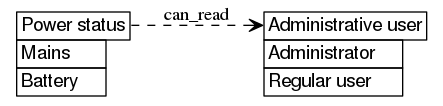Retrieving the value is denied if not administrative user

This sum does not support direct modification.

This sum can change as if by itself or indirectly because of other actions.

This sum can assume the following values:

 constant true Mains Unit is powered from at least one bus, outlets can be driven constant false Battery Unit is powered from the battery only, outlets cannot be driven, unit shutdown expected

Sample `uom` library read command:

```print(uom.dump(uom.meter.power_status))
```

```true
```

Sample `curl` read command:

```curl --digest -u admin:1234 -H "Accept: application/json" "http://192.168.0.100/restapi/meter/power_status/"
```

```true
```

# Emergency shutoff status

 sum (optional boolean) meter/emergency_off_status/ Status of emergency shutoff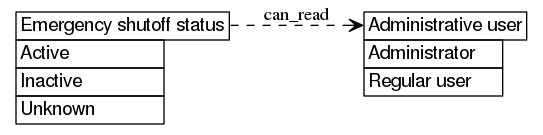Retrieving the value is denied if not administrative user

This sum does not support direct modification.

This sum can change as if by itself or indirectly because of other actions.

This sum can assume the following values:

 constant true Active Emergency shutoff active, all outlets off constant false Inactive Emergency shutoff inactive, normal outlet operation constant null Unknown Emergency shutoff is in an unknown state, probably due to power failure, all outlets likely off

Sample `requests` read command:

```print(requests.get('http://192.168.0.100/restapi/meter/emergency_off_status/',auth=auth,headers=headers).json())
```

```False
```

Sample `curl` read command:

```curl --digest -u admin:1234 -H "Accept: application/json" "http://192.168.0.100/restapi/meter/emergency_off_status/"
```

```false
```

# Synchronize meter data

 call meter/synchronize/ Saves meter data to persistent storage expressly (synchronization takes place periodically even without this call)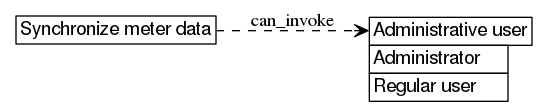Performing the function call is denied if not administrative user

The call has no arguments (supply empty tuple).

The call returns the following results:

1. Empty result: null

Sample JS `request` invocation:

```request.post({url:"meter/synchronize/",body:[]});
```

```(no output)
```

Sample `requests` invocation:

```requests.post('http://192.168.0.100/restapi/meter/synchronize/',auth=auth,headers=headers,json={})
```

```(no output)
```

## Empty result

 constant null meter/synchronize/results: 1/ No additional information available

This constant does not correspond to an actual resource.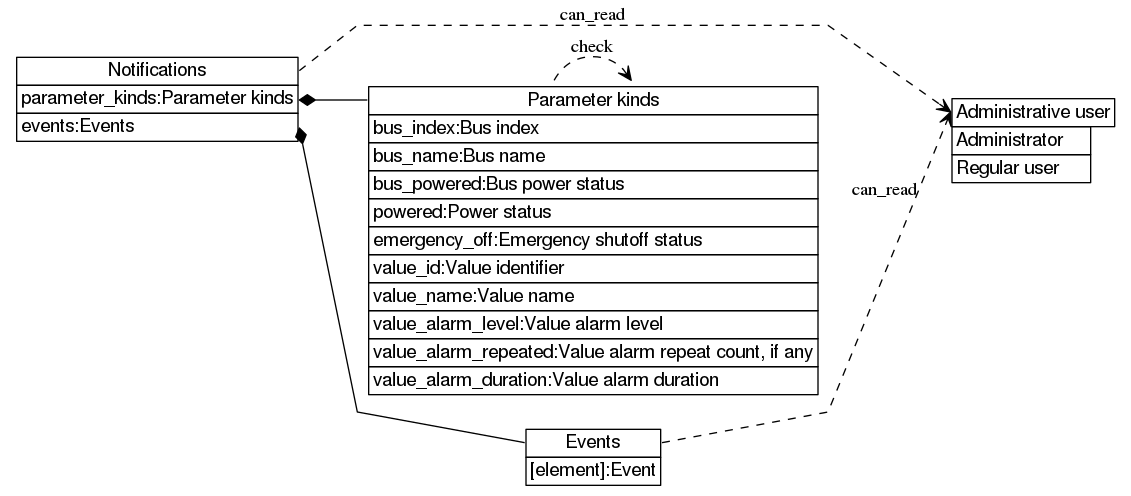Retrieving the value is denied if not administrative user

This object does not support direct modification.

This object contains the following items:

Sample `curl` read command:

```curl --digest -u admin:1234 -H "Accept: application/json" "http://192.168.0.100/restapi/meter/notifications/"
```

```...
```

Sample `uom` library read command:

```print(uom.dump(uom.meter.notifications))
```

```<...>
```

## Events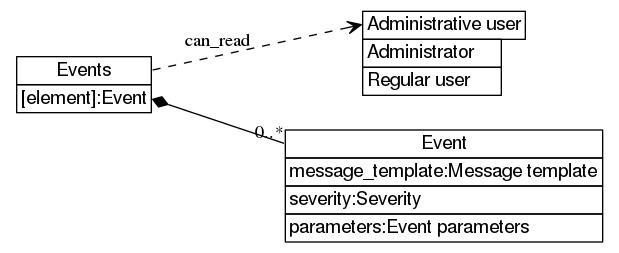Retrieving the value is denied if not administrative user

This map is composite, i.e. is modified as a whole.

This map does not support direct modification.

This map contains Event elements.

Creating elements in this collection is not supported.

Sample `uom` library read command:

```print(uom.dump(uom.meter.notifications.events))
```

```<...>
```

```uom get "meter/notifications/events"
```

```...
```

 object meter/notifications/parameter_kinds/ Kinds of event parameters

This object does not correspond to an actual resource.

This object contains the following items:

## Bus index

 number meter/notifications/parameter_kinds/bus_index/ Index of the bus

## Bus name

 number meter/notifications/parameter_kinds/bus_name/ Name of the bus

## Bus power status

 sum (boolean) meter/notifications/parameter_kinds/bus_powered/ Status of bus power

This sum can assume the following values:

 constant true Powered The bus is powered constant false Not powered The bus is not powered

## Power status

 sum (boolean) meter/notifications/parameter_kinds/powered/ Status of unit power

This sum can assume the following values:

 constant true Mains The unit is running on mains constant false Battery The unit is running on battery

## Emergency shutoff status

 sum (boolean) meter/notifications/parameter_kinds/emergency_off/ Status of emergency shutoff

This sum can assume the following values:

 constant true Active All outlets off constant false Inactive Normal outlet operation

## Value identifier

 string meter/notifications/parameter_kinds/value_id/ Identifier of the value

## Value name

 string meter/notifications/parameter_kinds/value_name/ Name of the value

## Value alarm level

 number meter/notifications/parameter_kinds/value_alarm_level/ Alarm level associated with the value

## Value alarm repeat count, if any

 sum (optional number) meter/notifications/parameter_kinds/value_alarm_repeated/ Number of consecutive times this alarm has been sent, if any

This sum can assume the following values:

 constant null Initial alarm The value has just entered the interval number Value alarm repeat count Number of consecutive times this alarm has been sent all of the following is true: the value is an integer the value is greater or equal to `0`

## Value alarm duration

 number meter/notifications/parameter_kinds/value_alarm_duration/ Amount of time the value has been at this alarm level

The value represents time, the standard unit of which is the second (s).

The value is subject to the following constraints: the value is greater or equal to `0`

# Event parameters: Event parameter

 object meter/notifications/events/S/parameters/N/ Properties of a parameter associated with the event

This object is a part of a composite value which is modified as a whole.

This object contains the following items:

Sample `requests` write command (note that `_event` and `_event_parameter_index` are variables):

```requests.put('http://192.168.0.100/restapi/meter/notifications/events/'+_event+'/parameters/'+str(_event_parameter_index)+'/',auth=auth,headers=headers,json={'fixed_value': False, 'has_fixed_value': True, 'id': 'powered'})
```

Sample JS `request` write command (note that `_event` and `_event_parameter_index` are variables):

```request.put({url:"meter/notifications/events/"+_event+"/parameters/"+_event_parameter_index.toString()+"/",body:{"fixed_value":false,"has_fixed_value":true,"id":"powered"}});
```

## Identifier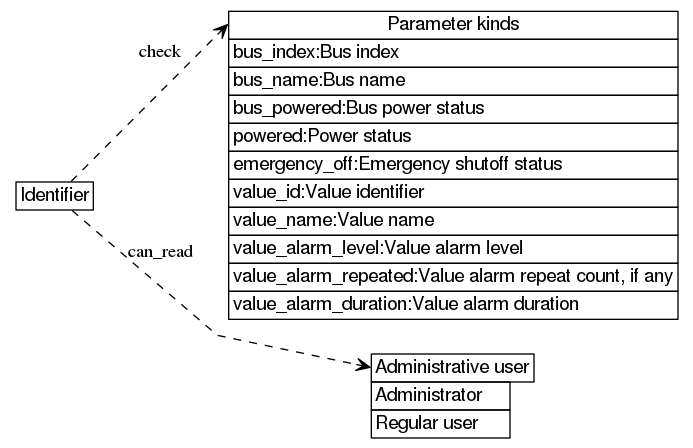The value is subject to the following constraints: parameter kinds[the value]

Retrieving the value is denied if not administrative user

This string is a part of a composite value which is modified as a whole.

This string does not support direct modification.

Sample `uom` library read command (note that `_event` and `_event_parameter_index` are variables):

```print(uom.dump(uom.meter.notifications.events[_event].parameters[_event_parameter_index].id))
```

```"emergency_off"
```

Sample `requests` read command (note that `_event` and `_event_parameter_index` are variables):

```print(requests.get('http://192.168.0.100/restapi/meter/notifications/events/'+_event+'/parameters/'+str(_event_parameter_index)+'/id/',auth=auth,headers=headers).json())
```

```'bus_index'
```

## Fixed value flag

 sum (boolean) meter/notifications/events/S/parameters/N/has_fixed_value/ Flag indicating that the parameter's value for this event is fixed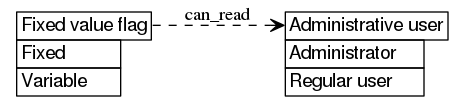Retrieving the value is denied if not administrative user

This sum is a part of a composite value which is modified as a whole.

This sum does not support direct modification.

This sum can assume the following values:

 constant true Fixed The value is fixed constant false Variable The value is variable

Sample `requests` read command (note that `_event` and `_event_parameter_index` are variables):

```print(requests.get('http://192.168.0.100/restapi/meter/notifications/events/'+_event+'/parameters/'+str(_event_parameter_index)+'/has_fixed_value/',auth=auth,headers=headers).json())
```

```True
```

Sample JS `request` read command (note that `_event` and `_event_parameter_index` are variables):

```request.get({url:"meter/notifications/events/"+_event+"/parameters/"+_event_parameter_index.toString()+"/has_fixed_value/"},function(error,response,body) {console.log(body)});
```

```false
```

## Fixed value

 sum (variant) meter/notifications/events/S/parameters/N/fixed_value/ Fixed parameter value for this particular event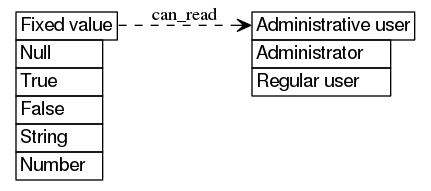Retrieving the value is denied if not administrative user

This sum is a part of a composite value which is modified as a whole.

This sum does not support direct modification.

This sum can assume the following values:

 constant null Null Null constant true True True constant false False False string String This string number Number This number

Sample `requests` read command (note that `_event` and `_event_parameter_index` are variables):

```print(requests.get('http://192.168.0.100/restapi/meter/notifications/events/'+_event+'/parameters/'+str(_event_parameter_index)+'/fixed_value/',auth=auth,headers=headers).json())
```

```None
```

Sample CLI read command (note that `event` and `event_parameter_index` are variables):

```uom get "meter/notifications/events/\${event}/parameters/\${event_parameter_index}/fixed_value"
```

```true
```

# Events: Event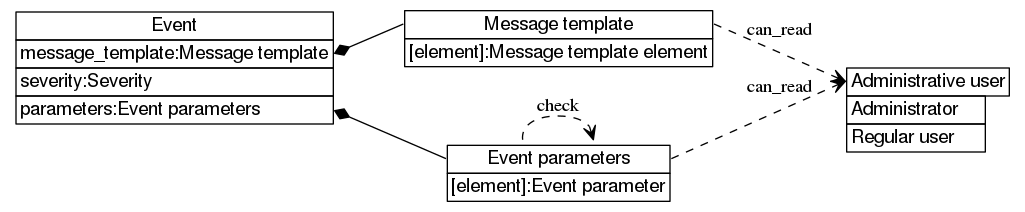This object is a part of a composite value which is modified as a whole.

This object contains the following items:

Sample JS `request` read command (note that `_event` is a variable):

```request.get({url:"meter/notifications/events/"+_event+"/"},function(error,response,body) {console.log(body)});
```

```{"severity":7,"message_template":["emergency shutoff status unknown due to power loss"],"parameters":[]}
```

Sample `requests` write command (note that `_event` is a variable):

```requests.put('http://192.168.0.100/restapi/meter/notifications/events/'+_event+'/',auth=auth,headers=headers,json={'severity': 5, 'message_template': ['running on battery'], 'parameters': [{'fixed_value': False, 'has_fixed_value': True, 'id': 'powered'}]})
```

## Message template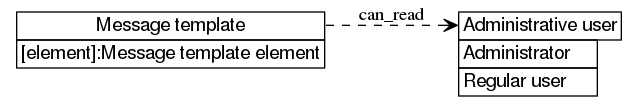Retrieving the value is denied if not administrative user

This array is a part of a composite value which is modified as a whole.

This array does not support direct modification.

This array contains Message template element elements.

Creating elements in this collection is not supported.

Sample `uom` library read command (note that `_event` is a variable):

```print(uom.dump(uom.meter.notifications.events[_event].message_template))
```

```{"emergency shutoff deactivated, normal outlet operation"}
```

Sample JS `request` read command (note that `_event` is a variable):

```request.get({url:"meter/notifications/events/"+_event+"/message_template/"},function(error,response,body) {console.log(body)});
```

```[0," is at alarm level ",1," for ",2," seconds"]
```

### Message template element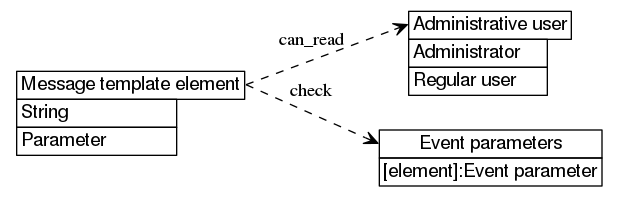Retrieving the value is denied if not administrative user

This sum is a part of a composite value which is modified as a whole.

This sum does not support direct modification.

This sum can assume the following values:

 string String Fixed string number Parameter Index of message parameter to insert event parameters[the value]

Sample `requests` read command (note that `_event` and `_message_template_element_index` are variables):

```print(requests.get('http://192.168.0.100/restapi/meter/notifications/events/'+_event+'/message_template/'+str(_message_template_element_index)+'/',auth=auth,headers=headers).json())
```

```'emergency shutoff deactivated, normal outlet operation'
```

Sample CLI read command (note that `event` and `message_template_element_index` are variables):

```uom get "meter/notifications/events/\${event}/message_template/\${message_template_element_index}"
```

```") is not powered"
```

## Severity

 sum (enumerated number) meter/notifications/events/S/severity/ Severity rating assigned to the event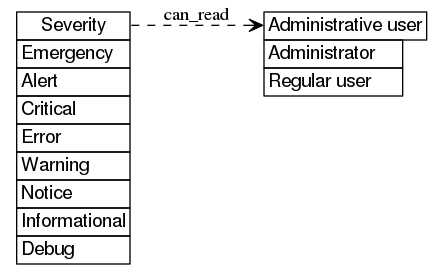Retrieving the value is denied if not administrative user

This sum is a part of a composite value which is modified as a whole.

This sum does not support direct modification.

This sum can assume the following values:

 constant 1 Emergency Treat the event as an emergency constant 2 Alert Treat the event as an alert constant 3 Critical Treat the event as critical constant 4 Error Treat the event as an error constant 5 Warning Treat the event as a warning constant 6 Notice Treat the event as a notice constant 7 Informational Treat the event as informational constant 8 Debug Treat the event as debugging-related

Sample JS `request` read command (note that `_event` is a variable):

```request.get({url:"meter/notifications/events/"+_event+"/severity/"},function(error,response,body) {console.log(body)});
```

```6
```

Sample `uom` library read command (note that `_event` is a variable):

```print(uom.dump(uom.meter.notifications.events[_event].severity))
```

```7
```

## Event parameters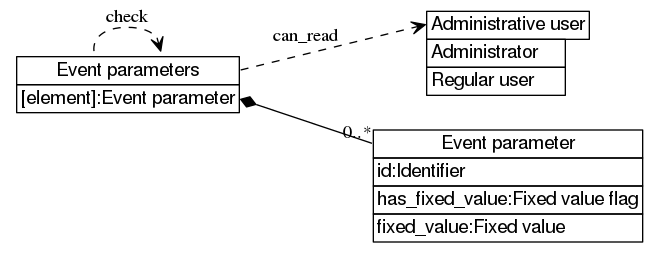Retrieving the value is denied if not administrative user

This array is a part of a composite value which is modified as a whole.

This array does not support direct modification.

This array contains Event parameter elements.

Creating elements in this collection is not supported.

Sample `curl` read command (note that `:event` is a URL template argument):

```curl --digest -u admin:1234 -H "Accept: application/json" "http://192.168.0.100/restapi/meter/notifications/events/:event/parameters/"
```

```[{"fixed_value":true,"has_fixed_value":true,"id":"powered"}]
```

Sample CLI read command (note that `event` is a variable):

```uom get "meter/notifications/events/\${event}/parameters"
```

```[{"fixed_value":true,"has_fixed_value":true,"id":"powered"}]
```